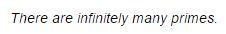# Infinitude of Primes - An Impossible InjectionThis is a recent proof by Dustin J. Mixon. (I am grateful to Claus Ivo Doering for bringing it to my attention.)

### Proof

Suppose there are a total of $N\;$ primes, $2=p_{1}\lt p_{2}\lt\ldots\lt p_{N}$, and pick $K\;$ such that $2^{K}\gt (K+1)^N$.

Consider the mapping $f: \{1,\ldots,2^{K}\}\rightarrow\{0,\ldots,K\}^N\;$ defined by $f(x):=(k_{1},\ldots,k_{N})$, where $x=p_{1}^{k_1}\cdot\ldots\cdot p_{N}^{k_N}\;$ is the prime factorization of $x$.

Here, the fact that $f(x)\in\{0,\ldots,K\}^N\;$ follows from

$\displaystyle K\ge\sum_{n=1}^{N}k_{n}\mbox{log}_{2}p_{n}\ge\sum_{n=1}^{N}k_{n}\ge \mbox{max}(k_{1},\ldots,k_{N}).$

Then $f\;$ is injective by the fundamental theorem of arithmetic, which contradicts the Pigeonhole Principle.

### Note

The proof above appears to be based on ideas already used by A. Thue in 1897.

### References

1. D. J. Mixon, Another Simple Proof of Infinitude of Primes, Am Math Monthly, 119 (2012), n 9, 811